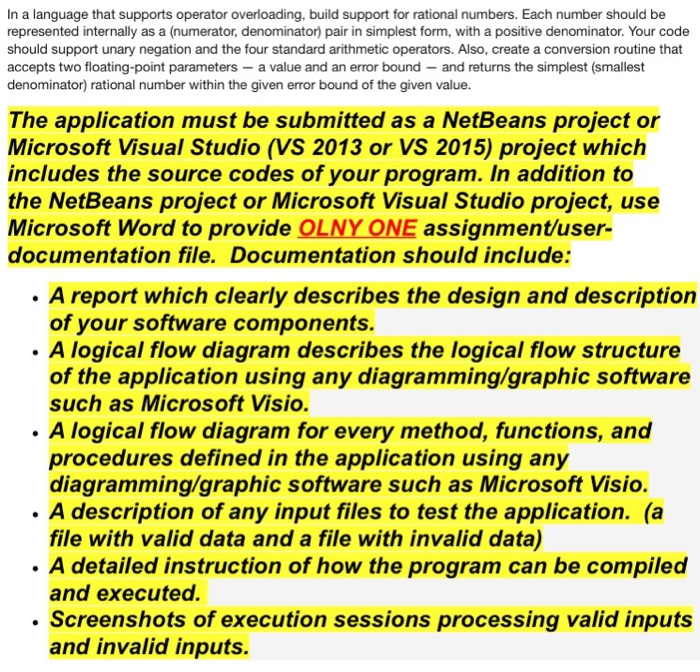# Answered Essay: In a language that supports operator overloading, build support for rational numbers. Each number should be represIn a language that supports operator overloading, build support for rational numbers. Each number should be represented internally as a (numerator, denominator) pair in simplest form, with a positive denominator. Your code should support unary negation and the four standard arithmetic operators. Also, create a conversion routine that accepts two floating-point parameters – a value and an error bound-and returns the simplest (smallest denominator) rational number within the given error bound of the given value. The application must be submitted as a NetBeans project or Microsoft Visual Studio (VS 2013 or VS 2015) project which includes the source codes of your program. In addition to the NetBeans project or Microsoft Visual Studio project, use Microsoft Word to provide OLNY ONE assignment/user-documentation file. Documentation should include: A report which clearly describes the design and description of your software components. A logical flow diagram describes the logical flow structure of the application using any diagramming/graphic software such as Microsoft Visio. A logical flow diagram for every method, functions, and procedures defined in the application using any diagramming/graphic software such as Microsoft Visio. A description of any input files to test the application. (a file with valid data and a file with invalid data). A detailed instruction of how the program can be compiled and executed. Screenshots of execution sessions processing valid inputs and invalid inputs.

//Rational.java
public class Rational
{

private int numerator;
private int denominator;

//constructors
Rational()
{
numerator = 0;
denominator = 1;
}
Rational(int num, int den)
{
numerator = num;
denominator = den;
}

//getters
public int getNumerator()
{
return numerator;
}

public int getDenominator()
{
return denominator;
}

//modifiers
public void setNumerator(int num)
{
numerator = num;
}

public void setDenominator(int den)
{
denominator = den;
}

public String toString()
{
return numerator + “/” + denominator;
}

private int gcd(int num, int den)
{
int r;
while(den != 0)
{
r = num % den;
num = den;
den = r;
}
return num;
}

{
int n;
int d;
n = (numerator * f.denominator) + (f.numerator * denominator);
d = denominator * f.denominator;
return new Rational(n/gcd(n, d),d/gcd(n, d));
}

//subtract 2 rational numbers
public Rational sub(Rational f)
{
int n;
int d;
n = (numerator * f.denominator)
– (f.numerator* denominator);
d= denominator * f.denominator;
return new Rational(n/gcd(n, d),d/gcd(n, d));
}

//multiply 2 rational numbers
public Rational mul(Rational f)
{
int n;
int d;
n = numerator * f.numerator;
d = denominator * f.denominator;
return new Rational(n/gcd(n, d),d/gcd(n, d));
}

public Rational div(Rational f)
{
int n;
int d;
n = numerator * f.denominator;
d = f.denominator * numerator;
return new Rational(n/gcd(n, d),d/gcd(n, d));
}
}

—————————————————————————————————————-

/**
* The java program TestRational that tests
* the methods of Rational class
* */
//TestRational.java
public class TestRational
{
public static void main(String[] args)
{
//create two objects of type Rational
Rational r1=new Rational(1, 2);
Rational r2=new Rational(1, 2);

//print to console
System.out.println(“Rational,r1 = ”
+r1.toString());
System.out.println(“Rational,r2 = ”
+r2.toString());

System.out.println(“Subtraction of two rational numbers”);
//calling sub method
System.out.println(r1.sub(r2));
System.out.println(“Multiplication of two rational numbers”);
//calling mul method
System.out.println(r1.mul(r2));
System.out.println(“Division of two rational numbers”);
//calling div method
System.out.println(r1.div(r2));
}
}

—————————————————————————————————————-

Sample Output:

Rational,r1 = 1/2
Rational,r2 = 1/2
1/1
Subtraction of two rational numbers
0/1
Multiplication of two rational numbers
1/4
Division of two rational numbers
1/1Pages (550 words)
Approximate price: -

Help Me Write My Essay - Reasons:Best Online Essay Writing Service

We strive to give our customers the best online essay writing experience. We Make sure essays are submitted on time and all the instructions are followed.Our Writers are Experienced and Professional

Our essay writing service is founded on professional writers who are on stand by to help you any time.Free Revision Fo all Essays

Sometimes you may require our writers to add on a point to make your essay as customised as possible, we will give you unlimited times to do this. And we will do it for free.Timely Essay(s)

We understand the frustrations that comes with late essays and our writers are extra careful to not violate this term. Our support team is always engauging our writers to help you have your essay ahead of time.Customised Essays &100% Confidential

Our Online writing Service has zero torelance for plagiarised papers. We have plagiarism checking tool that generate plagiarism reports just to make sure you are satisfied.Try it now!

## Calculate the price of your order

Total price:
\$0.00

How it works?Fill in the order form and provide all details of your assignment.Proceed with the payment

Choose the payment system that suits you most.HOW OUR ONLINE ESSAY WRITING SERVICE WORKS

Let us write that nagging essay.By clicking on the "PLACE ORDER" button, tell us your requires. Be precise for an accurate customised essay. You may also upload any reading materials where applicable.Pick A & Writer

Our ordering form will provide you with a list of writers and their feedbacks. At step 2, its time select a writer. Our online agents are on stand by to help you just in case.Editing (OUR PART)

At this stage, our editor will go through your essay and make sure your writer did meet all the instructions.